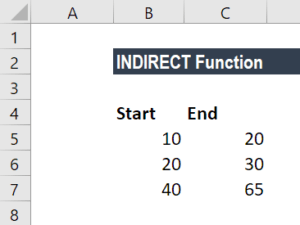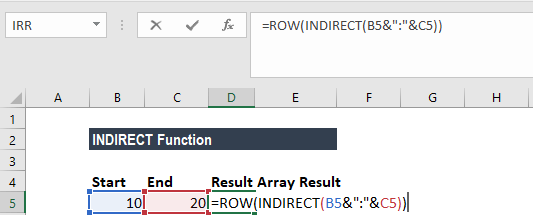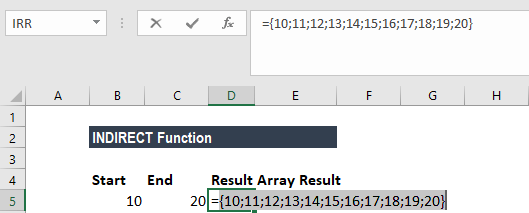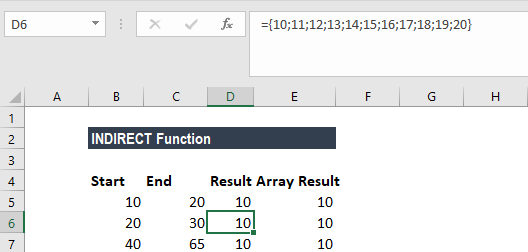# INDIRECT Function

Converts a text string into a cell reference

## What is the INDIRECT Function?

The INDIRECT function is categorized under Excel Lookup and Reference functions. It will convert a text string into a cell reference. For example, if we type the reference A1 in a formula, Excel will understand that it refers to cell A1. However, if the cell reference given takes the form of a text string, we need to use the INDIRECT function.

In financial analysis, the function is useful when we import data from an external source and we want to convert a reference assembled as text into a proper reference. In other words, the INDIRECT function helps us to create an Excel dynamic cell reference or range reference rather than manually entering the references into our Excel formulas.

### Formula

=INDIRECT(ref_text, [a1])

The INDIRECT function uses the following arguments:

1. Ref_text (required argument) – This is the text describing the reference.
2. a1 (optional argument) – This defines the style of the ref_text reference. It can be either TRUE (denotes that the reference is in A1 style) or FALSE (denotes that the reference is in R1C1 style).

When the a1 argument is omitted, it takes on the default value “TRUE.” When a1 is set to FALSE, INDIRECT will created an R1C1-style reference.

### How to use the INDIRECT Function in Excel?

To understand the uses of the function, let us consider an example:

#### Example 1

If we want to create an array of numbers like {10;20;30;40;50}, we can use a formula based on the ROW and INDIRECT functions. The technique can be used in array formulas that need a numeric array for processing of some kind.

Suppose we are given the following data:The formula to use is:After the formula is typed, we need to use F9 to see the array. We get the result below:Remember that when the formula is entered into a single cell, Excel will display only the first item in the array. As mentioned above, we need to use F9 in the formula bar to see the actual array result. Ideally, we use this formula inside a larger array formula, entered with the Control + Shift + Enter command.### Things to remember about the INDIRECT Function

1. #REF! error – Occurs if the given ref_text is not recognized as a valid cell reference.
2. By default, Excel uses the A1 referencing style. If we wish to use the R1C1 referencing style, we need to set this in the application’s settings.

Thanks for reading CFI’s guide to important Excel functions! By taking the time to learn and master these functions, you’ll significantly speed up your Excel modeling. To learn more, check out these additional CFI resources:

• Excel Functions for Finance